# IF and Then for ISACTUALVERSION and Forecast periodsHello,

I am working on Strategic Planning where I have actuals for 2019 and I am projecting for 2020, 2021, and 2022. I have a formula where I want to apply a 3% escalation starting in 2021.  My current formula uses ISACTUALVERSION and Offset.

Here is the formula: IF ISACTUALVERSION() THEN 'Actuals'.Period[SUM: 'Actuals'.AU Lookup] ELSE OFFSET('Actuals'.Period[SUM: 'Actuals'.AU Lookup], -12, 0) * Cost Escalation Factor.

The formula works for calculating 3% escalation for 2020 as it is able to pull actuals from 2019.  The current formula does not work for 2021 and 2022. How can I update the formula so that for 2021 it looks for what has been forecasted for 2020 and then applies the 3% escalation to that and also for 2022 it looks at what has been forecasted for 2021 and then applies the 3% escalation for that.

Thanks

Tagged:

•Make sure you are using offset on the same line item "\$ Amount Forecast - 3". Also, the ELSE should not be zero but rather the actual amount.

So something like this:

\$ Amount Forecast - 3 = IF NOT Is Actual Version? THEN OFFSET(\$ Amount Forecast - 3, -12, 0) * (1+Cost Escalation Factor) ELSE '\$ Amount Actuals'.

•@svbhagat that will work if your calendars are aligned for those extra years. Make sure you've got your model calendar or your time range appropriately set.

Another idea for you which is a little more aligned with the DISCO method is to create a system module that has all the annual increases in it. This cuts down on the number of calculations in your output module and improves performance.

Start by creating a time systems module like this: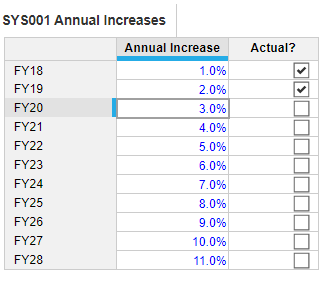Then change your formula to refer to your system module. Calculate once, refer often!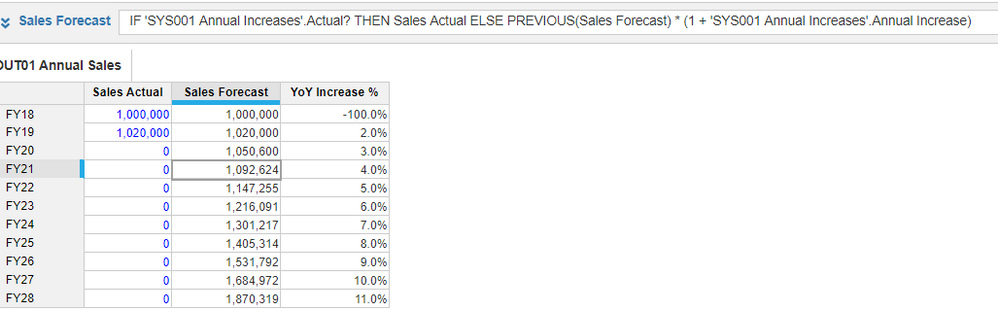•Hello @JaredDolich ,

Thank you for the suggestions.

My Time Settings do include the 3 out years 2020 through 2022.  Would you be able to help me understand what else could be missing in the current formula for 2021 and 2022 not to be calculating the 3% correctly?  Is there a way for me to update my current formula without building out the Time Setting system module you mentioned?

The Time Setting system module is definitely a good suggestion and something I learned about during the Level 1 Model Building training.  I do plan on setting that up for our models as we progress through.

Thanks

•@svbhagat can you take a screenshot of the time settings and a screenshot of your module blueprint?

Let's take a look at what's going on.

•Hello @JaredDolich ,

Here is a screenshot of my Time Settings.  We are currently not using Time Ranges.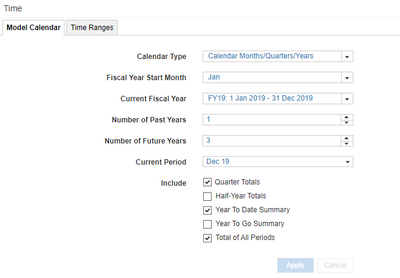Here is my blueprint for the calculation module:  Please note I had sent a simplified formula in my initial comments, but it is a longer formula below due to the different sum we have in the formula.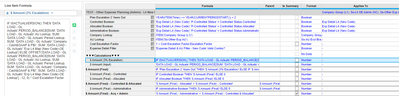•One more quick question before I try to replicate this. Is your module using the model calendar? You can see it in the module blueprint, scroll to the right a bit.

•Yes it is using the model calendar.  Please see revised screenshot.  Thanks.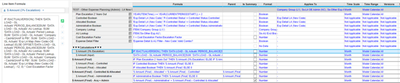•@svbhagat you were actually really close.

You  just need to change the offset to itself. Don't sum on actuals because it ends after 12 periods.

Solution below...

Some pointers, if I may be a little presumptuous:

- Use system modules: much more efficient both in space and in performance.

- Avoid using subsidiary views like the one you used for the 3%. Confusing, and doesn't meet the PLANS standard, particularly Auditable.

Here is the actual data (no versions, just actuals)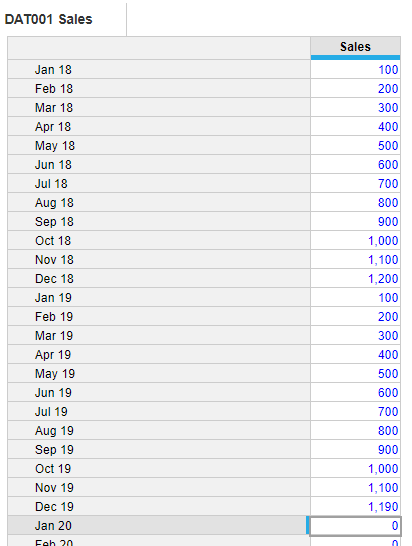If the time period is not an actual then look back on itself 12 periods and multiply times 1.03.

If the time period is an actual then give the actual.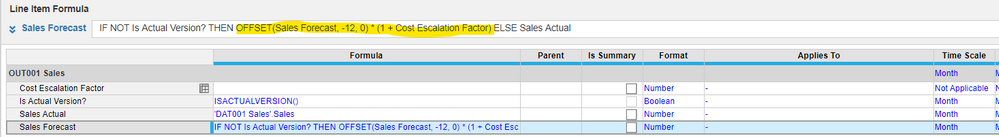This will produce the values you are looking for.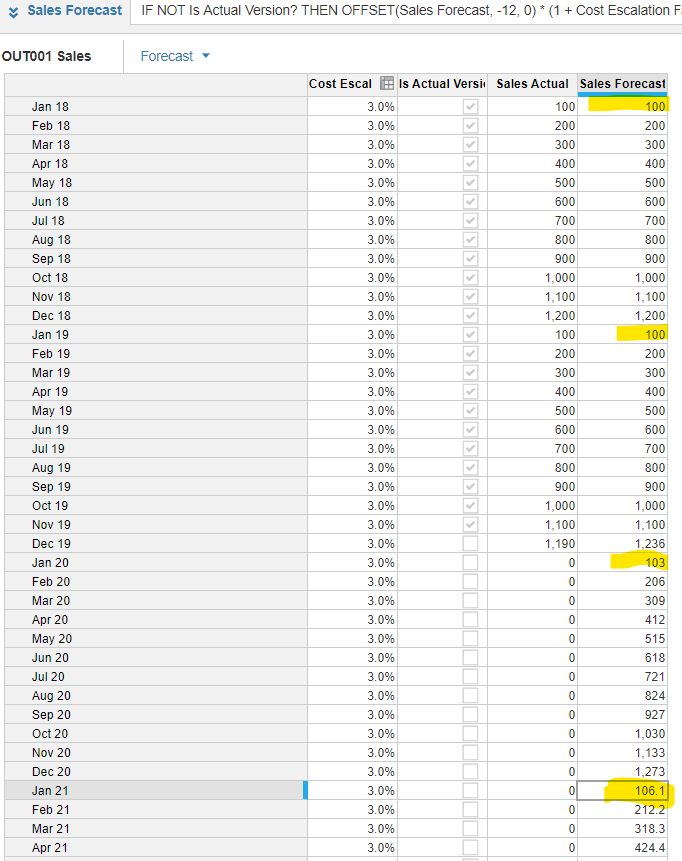•Hello @JaredDolich

Thank you for the detailed suggestions.  I've incorporated those formulas, but for some reason my FY 2021 and FY 2022 are still not calculating correctly.  Please see below: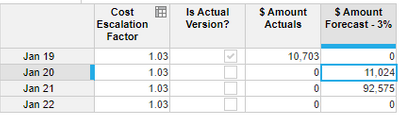Here is the formula I used for the \$ Amount Forecast - 3 = IF NOT Is Actual Version? THEN OFFSET('\$ Amount (Final)', -12, 0) * Cost Escalation Factor ELSE 0.

I'm not sure what else could be missing?

Thanks

•Thank you so much.  This was the solution I needed.  I missed the comment about using the same line item for the OFFSET.  That was it.

Appreciate all your help today.  I will reach out again if I have additional questions.

•Hello @JaredDolich ,

I'm working on a different formula in the same module.  Let me know if I should move this to a different post but it's related so I'm posting here.

I have three line items - 3% Escalation, Manual Input, Final.

The 3% Escalation is the formula you assisted with earlier.  The Manual Input line is to enter a different number if we don't want to use the 3% escalation.  The Final Line item is where it picks one or the other.

I need some assistance in figuring out the Final Formula for the forecast years.

For FY 2020 - We only want to use the Input Line.

For FY 2021 and FY 2022 - We want the formula to default to using 3% escalation unless the Input line is populated or we have identified that that item should be projected at \$0.

Is there any other way to incorporate this without having to setup a boolean for which line items we want to leave at \$0 vs. which ones we want to use 3% vs. which ones to use manual input?

The reason we would want to leave certain line items at \$0 is because they only occurred once or twice a year so no trends.

Here are the screenshots for the formula that I have been attempting but it is not accomplishing the results I would like to see: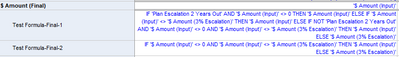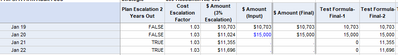Here is a screenshot of how I would like to see the results: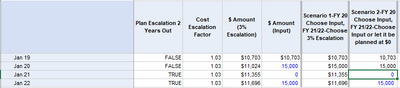Thank you

•Awesome question.

This can be done with complex nested if statements but the real answer is what you mentioned early on.

Create two system modules.

1. One for the Booleans that meet your conditions for rate increases.
2. One for the rate increases per year (which will also contain the alternative (0%) if one of your Booleans is tripped)

You will make your life SO much easier and also for those that need to support whatever it is  you build if you follow the DISCO method.

It's worth it. Trust me - I used complex, nested, if statements for so long. Once I went through the cert. level 2 course, it changed my perspective completely.

There's no going back...

If you want, we can carry this conversation offline. We can link up on LinkedIn - just search for Jared Dolich and I'll give you my contact info.

•Hello @JaredDolich

Thank you for your help.  I agree that building out the System Modules would be the right step but we are in a time crunch and I may not have the time and resources to complete that at this time.  Therefore I was looking for a formula I could apply to the existing module.

I look forward to connecting with you soon.  Thanks for your help.